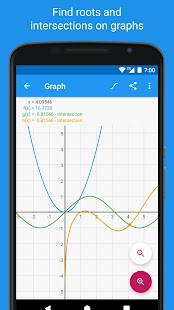# Graphing Calculator – Algeo | Free Plotting v2.33 [Pro] [Latest]

Report this app

## DescriptionUse Algeo as a physics calculator, a scientific calculator, an algebra calculator, and a chemistry calculator!

Algeo is the most beautiful scientific graphing calculator available on the Play Store. It’s fast and powerful and you’ll never have to carry around a large physical scientific calculator anymore. The intuitive interface shows your graphing calculation as you would write it on paper rather than squeezing everything on a single line. And you don’t need an internet connection unlike other graphing calculators! Useful for calculus, physics or as a chemistry calculator.
Wolfram Alpha users love using Algeo! This free app is packed with more features than a paid physics calculator. Graph fast with Algeo.

Draw functions, find intersections and show a table of values of the functions with an easy to use interface.

Use Algeo as a
• Graphing Calculator
• Physics Calculator
• Scientific Calculator
• Algebra Calculator
• Calculus Calculator
• Chemistry Calculator

As a calculus calculator
• Symbolic Differentiation
• Calculate Integrals (definite only)
• Calculate Taylor-series
• Solve Equations
• Algeo is the most advanced calculus calculator on the Google Play Store!

As a scientific calculator
• Trigonometric and Hyperbolic Functions
• Logarithm
• Result History
• Variables
• Scientific Notation
• Combinatorial functions
• Number theoretic functions (modulo, greatest common divisor)
• Use Algeo as your go-to algebra calculator!

As a graphing calculator
• Graph fast!
• Graph up to four functions
• Analyze function
• Find roots and intersections automatically
• Pinch to zoom
• Create a table of values for a function

This graphing calculator is the easiest way to analyze a function and graph fast.

Wolfram Alpha users approve! Algeo is the best scientific calculator for Wolfram Alpha users.

WHAT’S NEW

Greatly improved handling of singularities and discontinuities in plotting
Refined entry of divisions

Mod Info:

• Pro / Paid features unlocked;
• Disabled / Removed unwanted Permissions + Receivers + Providers + Services;
• Optimized and zipaligned graphics and cleaned resources for fast load;
• Ads Permissions / Services / Providers removed from Android.manifest;
• Google Play Store install package check disabled;
• Debug code removed;
• Remove default .source tags name of the corresponding java files;
• Analytics / Crashlytics / Firebase disabled;
• AOSP compatible mode;
• Languages: Full Multi Languages;
• CPUs: universal architecture;
• Screen DPIs: 120dpi, 160dpi, 240dpi, 320dpi, 480dpi, 640dpi;
• Original package signature changed.

Screenshots

•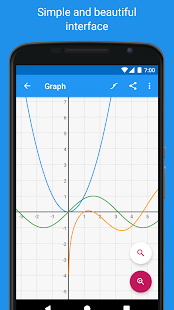•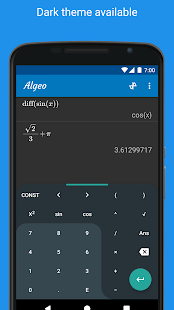•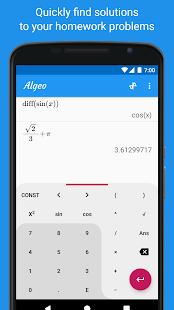•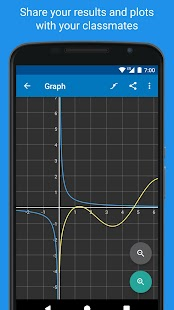•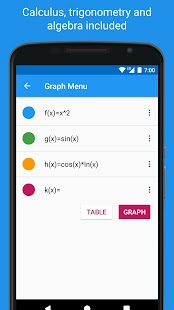•